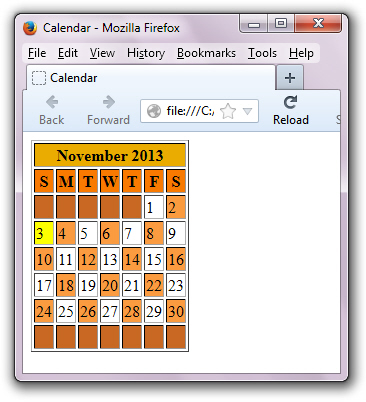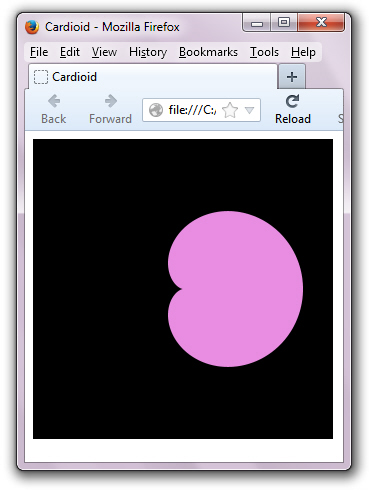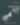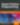# Augmenting JavaScript Core Objects Revisited

JavaScript

Share:

#### Free JavaScript Book!Write powerful, clean and maintainable JavaScript.

RRP \$11.95

My recent Augmenting JavaScript Core Objects article showed how to introduce new properties and methods to JavaScript’s `Array`, `Boolean`, `Date`, `Math`, `Number`, and `String` core objects. I followed in the tradition of other articles and blog posts, including those listed below, that show how to extend these core objects with new capabilities:

Directly adding properties to a core object or its prototype is controversial. In his Extending JavaScript Natives blog post, Angus Croll addresses several problems with this approach. For example, future browser versions may implement an efficient property or method that gets clobbered by a less efficient custom property/method. Read Croll’s blog post for more information on this and other problems.

Because core object augmentation is powerful and elegant, there should be a way to leverage this feature while avoiding its problems. Fortunately, there is a way to accomplish this task, by leveraging the adapter design pattern, which is also known as the wrapper pattern. In this article, I introduce a new version of my library that uses wrapper to augment various core objects without actually augmenting them.

## Exploring a New Core Object Augmentation Library

My new core object augmentation library attempts to minimize its impact on the global namespace by leveraging the JavaScript Module Pattern, which places all library code in an anonymous closure. This library currently exports `_Date` and `_Math` objects that wrap themselves around `Date` and `Math`, and is accessed by interrogating the `ca_tutortutor_AJSCOLib` global variable.

About `ca_tutortutor_AJSCOLib`
The `ca_tutortutor_AJSCOLib` global variable provides access to the augmentation library. To minimize the chance of a name collision with another global variable, I’ve prefixed `AJSCOLib` with my reversed Internet domain name.

Listing 1 presents the contents of my library, which is stored in an `ajscolib.js` script file.

```var ca_tutortutor_AJSCOLib =
(function()
{
var my = {};

var _Date_ = Date;

function _Date(year, month, date, hours, minutes, seconds, ms)
{
if (year === undefined)
this.instance = new _Date_();
else
if (month === undefined)
this.instance = new _Date_(year);
else
if (hours === undefined)
this.instance = new _Date_(year, month, date);
else
this.instance = new _Date_(year, month, date, hours, minutes, seconds,
ms);

this.copy =
function()
{
return new _Date_(this.instance.getTime());
};

this.getDate =
function()
{
return this.instance.getDate();
};

this.getDay =
function()
{
return this.instance.getDay();
};

this.getFullYear =
function()
{
return this.instance.getFullYear();
};

this.getHours =
function()
{
return this.instance.getHours();
};

this.getMilliseconds =
function()
{
return this.instance.getMilliseconds();
};

this.getMinutes =
function()
{
return this.instance.getMinutes();
};

this.getMonth =
function()
{
return this.instance.getMonth();
};

this.getSeconds =
function()
{
return this.instance.getSeconds();
};

this.getTime =
function()
{
return this.instance.getTime();
};

this.getTimezoneOffset =
function()
{
return this.instance.getTimezoneOffset();
};

this.getUTCDate =
function()
{
return this.instance.getUTCDate();
};

this.getUTCDay =
function()
{
return this.instance.getUTCDay();
};

this.getUTCFullYear =
function()
{
return this.instance.getUTCFullYear();
};

this.getUTCHours =
function()
{
return this.instance.getUTCHours();
};

this.getUTCMilliseconds =
function()
{
return this.instance.getUTCMilliseconds();
};

this.getUTCMinutes =
function()
{
return this.instance.getUTCMinutes();
};

this.getUTCMonth =
function()
{
return this.instance.getUTCMonth();
};

this.getUTCSeconds =
function()
{
return this.instance.getUTCSeconds();
};

this.getYear =
function()
{
return this.instance.getYear();
};

this.isLeap =
function()
{
var year = this.instance.getFullYear();
return (year % 400 == 0) || (year % 4 == 0 && year % 100 != 0);
};

_Date.isLeap =
function(date)
{
if (date instanceof _Date)
date = date.instance;
var year = date.getFullYear();
return (year % 400 == 0) || (year % 4 == 0 && year % 100 != 0);
};

this.lastDay =
function()
{
return new _Date_(this.instance.getFullYear(),
this.instance.getMonth() + 1, 0).getDate();
};

_Date.monthNames = ["January", "February", "March", "April", "May",
"June", "July", "August", "September", "October",
"November", "December"];

_Date.parse =
function(date)
{
if (date instanceof _Date)
date = date.instance;
return _Date_.parse(date);
};

this.setDate =
function(date)
{
if (date instanceof _Date)
date = date.instance;
this.instance.setDate(date);
};

this.setFullYear =
function(date)
{
if (date instanceof _Date)
date = date.instance;
this.instance.setFullYear(date);
};

this.setHours =
function(date)
{
if (date instanceof _Date)
date = date.instance;
this.instance.setHours(date);
};

this.setMilliseconds =
function(date)
{
if (date instanceof _Date)
date = date.instance;
this.instance.setMilliseconds(date);
};

this.setMinutes =
function(date)
{
if (date instanceof _Date)
date = date.instance;
this.instance.setMinutes(date);
};

this.setMonth =
function(date)
{
if (date instanceof _Date)
date = date.instance;
this.instance.setMonth(date);
};

this.setSeconds =
function(date)
{
if (date instanceof _Date)
date = date.instance;
this.instance.setSeconds(date);
};

this.setTime =
function(date)
{
if (date instanceof _Date)
date = date.instance;
this.instance.setTime(date);
};

this.setUTCDate =
function(date)
{
if (date instanceof _Date)
date = date.instance;
this.instance.setUTCDate(date);
};

this.setUTCFullYear =
function(date)
{
if (date instanceof _Date)
date = date.instance;
this.instance.setUTCFullYear(date);
};

this.setUTCHours =
function(date)
{
if (date instanceof _Date)
date = date.instance;
this.instance.setUTCHours(date);
};

this.setUTCMilliseconds =
function(date)
{
if (date instanceof _Date)
date = date.instance;
this.instance.setUTCMilliseconds(date);
};

this.setUTCMinutes =
function(date)
{
if (date instanceof _Date)
date = date.instance;
this.instance.setUTCMinutes(date);
};

this.setUTCMonth =
function(date)
{
if (date instanceof _Date)
date = date.instance;
this.instance.setUTCMonth(date);
};

this.setUTCSeconds =
function(date)
{
if (date instanceof _Date)
date = date.instance;
this.instance.setUTCSeconds(date);
};

this.toDateString =
function()
{
return this.instance.toDateString();
};

this.toISOString =
function()
{
return this.instance.toISOString();
};

this.toJSON =
function()
{
return this.instance.toJSON();
};

this.toLocaleDateString =
function()
{
return this.instance.toLocaleDateString();
};

this.toLocaleTimeString =
function()
{
return this.instance.toLocaleTimeString();
};

this.toString =
function()
{
return this.instance.toString();
};

this.toTimeString =
function()
{
return this.instance.toTimeString();
};

this.toUTCString =
function()
{
return this.instance.toUTCString();
};

_Date.UTC =
function(date)
{
if (date instanceof _Date)
date = date.instance;
return _Date_.UTC(date);
};

this.valueOf =
function()
{
return this.instance.valueOf();
};
}
my._Date = _Date;

var _Math = {};

var props = Object.getOwnPropertyNames(Math);
props.forEach(function(key)
{
if (Math[key])
_Math[key] = Math[key];
});

if (!_Math.GOLDEN_RATIO)
_Math.GOLDEN_RATIO = 1.61803398874;

if (!_Math.rnd || _Math.rnd.length != 1)
_Math.rnd =
function(limit)
{
if (typeof limit != "number")
throw "illegal argument: " + limit;

return Math.random() * limit | 0;
};

if (!_Math.rndRange || _Math.rndRange.length != 2)
_Math.rndRange =
function(min, max)
{
if (typeof min != "number")
throw "illegal argument: " + min;

if (typeof max != "number")
throw "illegal argument: " + max;

return Math.floor(Math.random() * (max - min + 1)) + min;
};

if (!_Math.toDegrees || _Math.toDegrees.length != 1)
_Math.toDegrees =
{
throw "illegal argument: " + radians;

return radians * (180 / Math.PI);
};

function(degrees)
{
if (typeof degrees != "number")
throw "illegal argument: " + degrees;

return degrees * (Math.PI / 180);
};

if (!_Math.trunc || _Math.trunc.length != 1)
_Math.trunc =
function(n)
{
if (typeof n != "number")
throw "illegal argument: " + n;

return (n >= 0) ? Math.floor(n) : -Math.floor(-n);
};
my._Math = _Math;

return my;
}());```

Listing 1: This self-contained augmentation library can be extended to support all core objects

All variables and functions declared within the anonymous closure are local to that closure. To be accessed from outside the closure, a variable or function must be exported. To export the variable or function, simply add it to an object and return that object from the closure. In Listing 1, the object is known as `my` and is assigned a `_Date` function reference and a `_Math` object reference.

Following the declaration of variable `my`, which is initialized to an empty object, Listing 1 declares variable `_Date_`, which references the `Date` core object. Wherever I need to access `Date` from within the library, I refer to `_Date_` instead of `Date`. I’ll explain my reason for this arrangement later in this article.

Listing 1 now declares a `_Date` constructor for constructing `_Date` wrapper objects. This constructor declares the same `year`, `month`, `date`, `hours`, `minutes`, `seconds`, and `ms` parameters as the `Date` core object. These parameters are interrogated to determine which variant of the `Date` constructor to invoke:

• `_Date()` invokes `Date()` to initialize a `Date` object to the current date. This scenario is detected by testing `year` for `undefined`.
• `_Date(year)` invokes `Date(milliseconds)` or `Date(dateString)` to initialize a `Date` object to the specified number of milliseconds or date string — I leave it to `Date` to handle either case. This scenario is detected by testing `month` for `undefined`.
• `_Date(year, month, date)` invokes `_Date(year, month, date)` to initialize a `Date` object to the specified year, month, and day of month (date). This scenario is detected by testing `hour` for `undefined`.
• `_Date(year, month, day, hours, minutes, seconds, milliseconds)` invokes `Date(year, month, day, hours, minutes, seconds, milliseconds)` to initialize a `Date` object to the date described by the individual components. This scenario is the default.

Regardless of which constructor variant (a constructor invocation with all or fewer arguments) is invoked, the returned result is stored in `_Date`‘s `instance` property. You should never access `instance` directly because you may need to rename this property should `Date` introduce an `instance` property in the future. Not accessing `instance` outside of the library reduces code maintenance.

At this point, Listing 1 registers new `copy()`, `isLeap()`, and `lastDay()` methods, and a new `monthNames` property with `_Date`. It also registers `Date`‘s methods. The former methods augment `Date` with new functionality that’s associated with `_Date` instead of `Date`, and are described below. The latter methods use `instance` to access the previously stored `Date` instance, usually to invoke their `Date` counterparts:

• `copy()` creates a copy of the instance of the `Date` object that invokes this method. In other words, it clones the `Date` instance. Example: `var d = new Date(); var d2 = d.copy();`
• `isLeap()` returns true when the year portion of the invoking `Date` object instance represents a leap year; otherwise, false returns. Example: `var d = new Date(); alert(d.isLeap());`
• `isLeap(date)` returns true when the year portion of `date` represents a leap year; otherwise, false returns. Example: `alert(Date.isLeap(new Date()));`
• `lastDay()` returns the last day in the month of the invoking `Date` object instance. Example: `var d = new Date(); alert(d.lastDay());`
• Although not a method, you can obtain an English-based long month name from the `Date.monthNames` array property. Pass an index ranging from 0 through 11. Example: `alert(Date.monthNames)`

Methods that are associated with `_Date` instead of its instances are assigned directly to `_Date`, as in `_Date.UTC = function(date)`. The `date` parameter identifies either a core `Date` object reference or a `_Date` reference. Methods that are associated with `_Date` instances are assigned to `this`. Within the method, the `Date` instance is accessed via `this.instance`.

You would follow the previous protocol to support `Array`, `String`, and the other core objects — except for `Math`. Unlike the other core objects, you cannot construct `Math` objects. Instead, `Math` is simply a placeholder for storing static properties and methods. For this reason, I treat `Math` differently by declaring a `_Math` variable initialized to the empty object and assigning properties and methods directly to this object.

The first step in initializing `_Math` is to invoke `Object`‘s `getOwnPropertyNames()` method (implemented in ECMAScript 5 and supported by modern desktop browsers) to return an array of all properties (enumerable or not) found directly upon the argument object, which is `Math`. Listing 1 then assigns each property (function or otherwise) to `_Math` before introducing new properties/methods (when not already present):

• `GOLDEN_RATIO` is a constant for the golden ratio that I mentioned in my previous article. Example: `alert(Math.GOLDEN_RATIO);`
• `rnd(limit)` returns an integer ranging from 0 through one less than `limit`‘s value. Example: `alert(Math.rnd(10));`
• `rndRange(min, max)` returns a random integer ranging from `min`‘s value through `max`‘s value. Example: `alert(Math.rndRange(10, 20));`
• `toDegrees(radians)` converts the `radians` value to the equivalent value in degrees and returns this value. Example: `alert(Math.toDegrees(Math.PI));`
• `toRadians(degrees)` converts the `degrees` value to the equivalent value in radians and returns this value. Example: `alert(Math.toRadians(180));`
• `trunc(n)` removes the fractional part from the positive or negative number passed to `n` and returns the whole part. Example: `alert(Math.trunc(5.8));`

Each method throws an exception signifying an illegal argument when it detects an argument that’s not of `Number` type.

Why bother creating an augmentation library instead of creating separate utility objects (such as `DateUtil` or `MathUtil`)? The library serves as a massive shim to provide consistent functionality across browsers. For example, Firefox 25.0’s `Math` object exposes a `trunc()` method whereas this method is absent from Opera 12.16. My library ensures that a `trunc()` method is always available.

## Testing and Using the New Core Object Augmentation Library

Now that you’ve had a chance to explore the library, you’ll want to try it out. I’ve created a pair of scripts that test various new `_Date` and `_Math` capabilities, and have created a pair of more practical scripts that use the library more fully. Listing 2 presents an HTML document that embeds a script for testing `_Date`.

```<!DOCTYPE html>
<html>
<title>
Augmented Date Tester
</title>

<script type="text/javascript" src="ajscolib.js">
</script>

<body>
<script>
var Date = ca_tutortutor_AJSCOLib._Date;

var date = new Date();
var dateCopy = date.copy();
alert("Copy of current date: " + date.toString());
alert("Current date == Copy of current date: " + (date == dateCopy));
alert("Isleap " + date.toString() + ": " + date.isLeap());
alert("Isleap July 1, 2012: " + Date.isLeap(new Date(2012, 6, 1)));
</script>
</body>
</html>```

Listing 2: Testing the “augmented” `Date` object

When you work with this library, you won’t want to specify `ca_tutortutor_AJSCOLib._Date` and probably won’t want to specify `_Date`. Instead, you’ll want to specify `Date` as if you’re working with the core object itself. You shouldn’t have to change your code to change `Date` references to something else. Fortunately, you don’t have to do that.

The first line in the script assigns `ca_tutortutor_AJSCOLib._Date` to `Date`, effectively removing all access to the `Date` core object. This is the reason for specifying `var _Date_ = Date;` in the library. If I referred to `Date` instead of `_Date_` in the library code, you would observe “too much recursion” (and probably other problems).

The rest of the code looks familiar to those who’ve worked with `Date`. However, there’s a small hiccup. What gets output when you invoke `alert("Current date: " + date);`? If you were using the `Date` core object, you would observe `Current date: ` followed by a string representation of the current date. In the current context, however, you observe `Current date: ` followed by a numeric milliseconds value.

`toString()` versus `valueOf()`
Check out Object-to-Primitive Conversions in JavaScript to learn why `alert("Current date: " + date);` results in a string or numeric representation of `date`.

Let’s put the “augmented” `Date` object to some practical use, such as creating a calendar page. The script will use `document.writeln()` to output this page’s HTML based on the `<table>` element. Two variants of the `_Date` constructor along with the `getFullYear()`, `getMonth()`, `getDay()`, `lastDay()`, and `getDate()` methods, and the `monthNames` property will be used. Check out Listing 3.

```<!DOCTYPE html>
<html>
<title>
Calendar
</title>

<script type="text/javascript" src="ajscolib.js">
</script>

<body>
<script>
var Date = ca_tutortutor_AJSCOLib._Date;

var date = new Date();
var year = date.getFullYear();
var month = date.getMonth();
document.writeln("<table border=1>");
document.writeln("<th bgcolor=#eeaa00 colspan=7>");
document.writeln("<center>" + Date.monthNames[month] + " " + year +
"</center>");
document.writeln("</th>");
document.writeln("<tr bgcolor=#ff7700>");
document.writeln("<td><b><center>S</center></b></td>");
document.writeln("<td><b><center>M</center></b></td>");
document.writeln("<td><b><center>T</center></b></td>");
document.writeln("<td><b><center>W</center></b></td>");
document.writeln("<td><b><center>T</center></b></td>");
document.writeln("<td><b><center>F</center></b></td>");
document.writeln("<td><b><center>S</center></b></td>");
document.writeln("</tr>");
var dayOfWeek = new Date(year, month, 1).getDay();
var day = 1;
for (var row = 0; row < 6; row++)
{
document.writeln("<tr>");
for (var col = 0; col < 7; col++)
{
var row;
if ((row == 0 && col < dayOfWeek) || day > date.lastDay())
{
document.writeln("<td bgcolor=#cc6622>");
document.writeln(" ");
}
else
{
if (day == date.getDate())
document.writeln("<td bgcolor=#ffff00>");
else
if (day % 2 == 0)
document.writeln("<td bgcolor=#ff9940>");
else
document.writeln("<td>");
document.writeln(day++);
}
document.writeln("</td>");
}
document.writeln("</tr>");
}
document.writeln("</table>");
</script>
</body>
</html>```

Listing 3: Using the “augmented” `Date` object to generate a calendar page

To create a realistic calendar page, we need to know on which day of the week the first day of the month occurs. Expression `new Date(year, month, 1).getDay()` gives us the desired information (0 for Sunday, 1 for Monday, and so on), which is assigned to `dayOfWeek`. Every square on the top row whose column index is less than `dayOfWeek` is left blank.

Figure 1 shows a sample calendar page.Figure 1: The current day is highlighted in yellow.

Listing 4 presents an HTML document that embeds a script for testing `_Math`.

```<!DOCTYPE html>
<html>
<title>
Augmented Math Tester
</title>

<script type="text/javascript" src="ajscolib.js">
</script>

<body>
<script>
var Math = ca_tutortutor_AJSCOLib._Math;

try
{
}
catch (err)
{
}

for (var i = 0; i < 10; i++)

try
{
}
catch (err)
{
}

try
{
}
catch (err)
{
}

try
{
}
catch (err)
{
}
</script>
</body>
</html>```

Listing 4: Testing the “augmented” `Math` object

Let’s put the “augmented” `Math` object to some practical use, such as displaying a cardioid curve, which is a plane curve traced by a point on the perimeter of a circle that’s rolling around a fixed circle of the same radius. The script will use `Math`‘s `rndRange()`, `toRadians()`, `cos()`, and `sin()` methods. Check out Listing 5.

```<!DOCTYPE html>
<html>
<title>
Cardioid
</title>

<script type="text/javascript" src="ajscolib.js">
</script>

<body>
<canvas id="canvas" width="300" height="300">
canvas not supported
</canvas>

<script>
var Math = ca_tutortutor_AJSCOLib._Math;

var canvas = document.getElementById("canvas");
var canvasctx = canvas.getContext("2d");

var width = document.getElementById("canvas").width;
var height = document.getElementById("canvas").height;

canvasctx.fillStyle = "#000";
canvasctx.fillRect(0, 0, width, height);
canvasctx.fillStyle = "RGB(" + Math.rndRange(128, 255) + "," +
Math.rndRange(128, 255) + "," +
Math.rndRange(128, 255) + ")";

canvasctx.beginPath();
for (var angleDeg = -180.0; angleDeg < 180.0; angleDeg += 0.1)
{

// Evaluate cardioid curve equation. This produces radius for
// given angle. Note: [r, angle] are the polar coordinates.

var r = 60.0 + 60.0 * Math.cos(angle);

// Convert polar coordinates to rectangular coordinates. Add
// width / 2 and height / 2 to move curve's origin to center
// of canvas. (Origin defaults to canvas's upper-left corner.)

var x = r * Math.cos(angle) + width / 2;
var y = r * Math.sin(angle) + height / 2;
if (angle == 0.0)
canvasctx.moveTo(x, y);
else
canvasctx.lineTo(x, y)
}
canvasctx.closePath();
canvasctx.fill();
</script>
</body>
</html>```

Listing 5: Using the “augmented” `Math` object to generate a cardioid curve

Listing 5 uses HTML5’s canvas element and API to present the cardioid curve, which is constructed as a polygon via the canvas context’s `beginPath()`, `moveTo()`, `lineTo()`, and `closePath()` methods. Each component of the curve’s fill color is randomly chosen via `rndRange()`. Its arguments ensure that the component isn’t too dark. The curve is filled via the canvas context’s `fill()` method.

Figure 2 shows a colorful cardioid curve.Figure 2: Reload the page to change the curve’s color.

## Conclusion

This article showed how to create a library that augments JavaScript’s core objects without augmenting them directly. The library’s public interface is portable across browsers, although it’s possible that the implementation might need adjusting for compatibility, performance, or other reasons. As an exercise, add my previous augmentation article’s `Array`, `Boolean`, `Number`, and `String` enhancements to this library.### Jeff Friesen

Jeff Friesen is a freelance tutor and software developer with an emphasis on Java and mobile technologies. In addition to writing Java and Android books for Apress, Jeff has written numerous articles on Java and other technologies for SitePoint, InformIT, JavaWorld, java.net, and DevSource.

#### New books out now!Learn how Git works, and how to use it to streamline your workflow!Google, Netflix and ILM are Python users. Maybe you should too?